# Practice MCQs on Solid State Class 12 Chemistry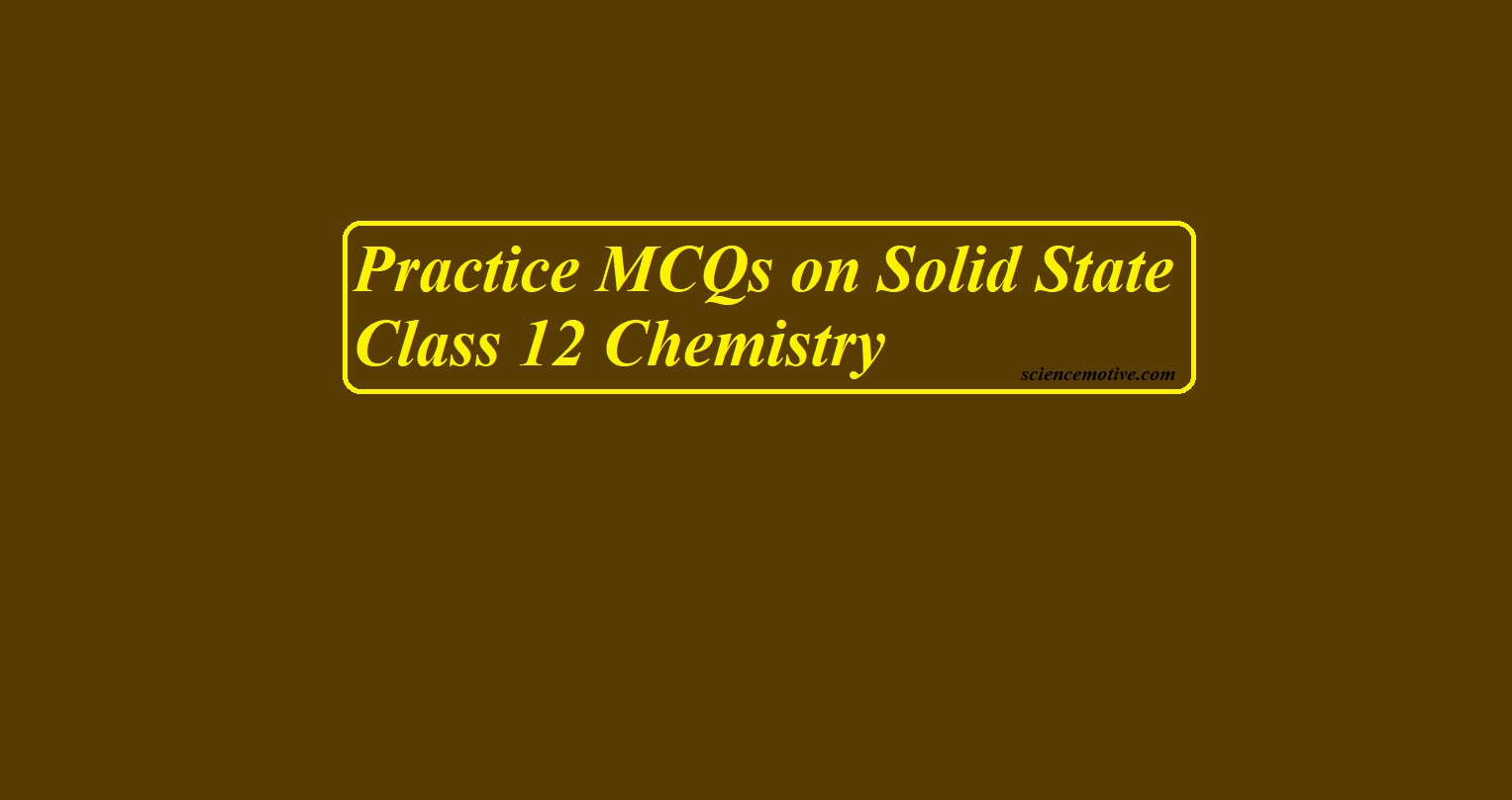# Practice MCQs on Solid State Class 12 Chemistry

Practice MCQs on Solid State Class 12 Chemistry

To get answers click the link given at the end of the post

1. Which of the following are not properties of solid?
(a) They have definite mass, volume, and shape
(b) Intermolecular forces are weak
(c) Intermolecular distances are short
(d) They are incompressible and rigid

2. Amorphous solids are
(a) Solid substance in real sense           (b) Liquid in the real sense
(c) Supercooled liquids                         (d) Substances with a definite melting point

3. Which of the following is not a crystalline solid?
(a) Common salt          (b) Sugar         (c) Iron            (d) Rubber

4. Solid carbon dioxide is an example of
(a) Ionic crystal                                   (b) Covalent crystal
(c) Metallic crystal                              (d) Molecular crystal

5. In a simple cubic unit cell, an atom at the corner contributes to the unit cell
(a) 1 part         (b) 1/2 part      (c) 1/4 part      (d) 1/8 part

6. The vacant space in the bcc unit cell is
(a) 32 %           (b) 23 %           (c) 26 %           (d) None of these

7. If an atom is present at the center of the cube, the contribution of that atom per unit cell
(a) ¼                (b) 1                 (c) ½                (d) 1/8

8. What is the coordination number in the hcp lattice
(a) 4                 (b) 6                 (c) 10               (d) 12

9. In an fcc unit cell, the contribution from the atom at the corner and face center of the cube are respectively
(a) 1/4, ½         (b) 1/8, ¼         (c) 1/8, ½         (d) 1/2, 1/2

10. The octahedral voids in fcc or ccp are located at
(a) Edge centres and along diagonals               (b) Edge centres and body centre
(c) Along body diagonal and edge centre         (c) All the edge centres only

11. In a face-centered lattice of X atoms, Y atoms occupy 1/2 of tetrahedral voids and 1/4 of octahedral voids. What is the possible formula of the compound?
(a) X4Y6          (b) X4Y5          (c) X4Y8          (d) X4Y9

12. In a face-centered lattice of X and Y, X atoms are present at the corners while Y atoms are at face centers. Then the formula of the compound is:
(a) XY3                        (b) X2Y3          (c) X3Y                        (d) XY

13. A compound contains P and Q elements. Atoms Q is in ccp arrangement while P occupies all tetrahedral sites. The formula of the compound is:
(a) PQ              (b) PQ2            (c) P2Q            (d) P3Q

14. In a closest packed lattice, the no. Of octahedral sites, as compared to tetrahedral ones will be
(a) Equal          (b) Half            (c) Double       (d) None of these

15. The correct order of the packing efficiency in different types of the unit cell is
(a) Fcc< bcc < simple cubic                 (b) Fcc> bcc > simple cubic
(c) Fcc< bcc > simple cubic                 (d) Bcc <fcc> simple cubic

Practice MCQs on Solid State Class 12 Chemistry

16. What is the relation between edge length (a) and radius (r) in a simple cubic lattice
(a) A = 4r        (b) A = r          (c) A = 2r        (d) A = r/2

17. Types of Bravais lattices in 3D arrangement is
(a) 8                 (b) 12               (c) 14               (d) 9

18. What is the packing efficiency of a simple cubic lattice
(a) 52%            (b) 74%           (c) 68%            (d) None of these

19. Patterns of layers in ccp is
(a) ABAB type                        (b) ABCABC type
(c) ABBA type                        (d) Abcbac type

20. The no. of octahedral voids per sphere in an fcc lattice is
(a) 8                 (b) 4                 (c) 2                 (d) 1

21. Following diagram represents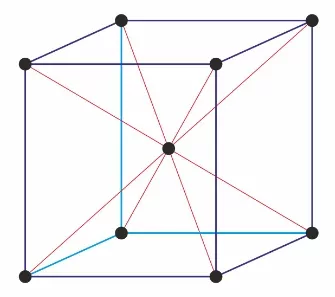(a) Simple cubic unit cell                      (b) Bcc
(c) Fcc                                                   (d) End centered

22. In the following diagrams, X and Y represent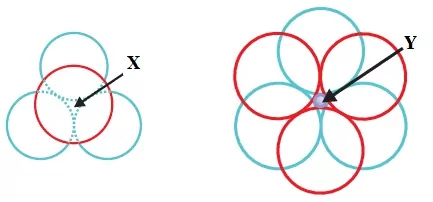(a) Tetrahedral void, triangular void     (b) Tetrahedral void, octahedral void
(c) Octahedral void, tetrahedral void    (d) Triangular void, octahedral void

23. Calculate the number of atoms in a cubic-based unit cell having one atom on each corner and two atoms on each body diagonal.
(a) 8                 (b) 16               (c) 9                 (d) 1

24. What is the two-dimensional coordination number of a molecule in a square close-packed layer?
(a) 6                 (d) 12               (c) 2                 (d) 4

25. Silver forms ccp lattice and X-ray studies of its crystals show that the edge length of its unit cell is 408.6 pm. Calculate the density of silver in gram per cubic cm(Atomic mass = 107.9 u).
(a) 11.5            (b) 10.5            (c) 1.05            (d) 105

Practice MCQs on Solid State Class 12 Chemistry

26. In the Schottky defect-
(a) Cations are missing from the lattice and occupy the interstitial sites
(b) Equal number of cations and anions are missing
(c) Anions are missing and electrons are present in their places
(d) Equal number of extra cations and electrons are present in the interstitial sites

27. As a result of the Schottky defect
(a) There is no effect on the density     (b) Density of the crystal increases
(c) Density of the crystal decreases      (d) Any of the above three can happen

28. F-centres in an ionic crystal is
(a) Lattice sites containing electrons    (b) Interstitial sites containing electrons
(c) Lattice sites that are vacant             (c) Interstitial sites containing cations

29. Doping of Si with P or Al increases the conductivity the difference in the two cases is
(a) P gives rise to extra electrons while Al gives rise to holes
(b) P gives rise to holes while Al gives rise to extra electrons
(c) P is metal whereas Al is a non-metal
(d) P is conductor while Al is a semiconductor

30. In a Solid lattice, the cation has left a lattice site and is located at an interstitial position, the lattice defect is
(a) Interstitial defect    (b) Vacancy defect   (c) Frenkel defect    (c) Schottky defect

31. Which of the following statements is not true?
(a) Paramagnetic substances are weakly attracted by the magnetic field
(b) Ferromagnetic substances cannot be magnetized permanently
(c) The domains in antiferromagnetic substances are oppositely oriented with respect to each other
(d) Pairing of electrons cancels their magnetic moment in the diamagnetic substances

32. A ferromagnetic substance becomes a permanent magnet when placed in a magnetic field because—
(a) All the domains get oriented in the direction of the magnetic field.
(b) All the domains get oriented in the direction opposite to the direction of the magnetic field
(c) Domains get oriented randomly
(d) Domains are not affected by the magnetic field

33. Which of the following defects is also known as a dislocation defect?
(a) Frenkel defect                                  (b) Schottky defect
(c) Non-stoichiometric defect               (d) Simple interstitial defect

34. Which type of defect has the presence of cations in the interstitial sites?
(a) Frenkel defect                                  (b) Schottky defect
(c) Metal deficiency defect                   (d) Vacancy defect

35. The appearance of colour in solid alkali metal halide is generally due to:
(a) Frenkel defect                                 (b) Schottky defect
(c) Vacancy defect                                (d) F-Centres

Practice MCQs on Solid State Class 12 Chemistry

36. Compound that can exhibit Frenkel, as well as Schottky defect, is
(a) AgCl                       (b) AgBr                      (c) NaCl                       (d) ZnS

37. A group 14 element is to be converted into an n-type semiconductor by doping it with suitable impurity. To which group should this impurity belong?
(a) Group 13    (b) Group 14    (c) Group 15    (d) Group 12

38. What happens when Fe3O4 is heated at 850 K?
(a) It converts into a diamagnetic substance
(b) It converts into a ferromagnetic substance
(c) It converts into a paramagnetic substance
(d) No effect

39. What is the effect of increasing temperature on the electrical conductivity of metals
(a) Increases    (b) Decrease        (c) No change           (d) Can’t predicted

40. The difference in the electrical conductivity of metals, insulators, and semiconductors can be understood in terms of
(a) VBT           (b) Band theory           (c) Resonance              (d) Hybridization

41. Non-stoichiometric Metal Excess defects due to interstitial cations are found in
(a) NaCl           (b) ZnO            (c) ZnS            (d) AgBr

42. What type of defect can arise when a solid is heated?
(a) Vacancy defect                                (b) Interstitial defect
(c) Metal excess defect                         (d) Impurity defect

43. Which compound is added to create an impurity defect in NaCl?
(a) AgBr          (b) AgCl          (c) KI               (d) SrCl2

Assertion and reason both are correct statements and reason is the correct explanation for assertion.
Assertion and reason both are correct statements but the reason is not a correct explanation for assertion.
The assertion is a correct statement but the reason is the wrong statement.
The assertion is a wrong statement but the reason is the correct statement.

44. Assertion: Total number of octahedral voids present in the unit cell of cubic close packing including the one that is present at the body centre, is four.
Reason: Besides the body centre there is one octahedral void present at the centre of each of the six faces of the unit cell and each of which is shared between two adjacent unit cells.

45. Following diagram represents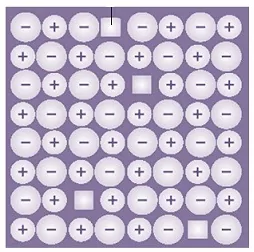(a) Frenkel defects                   (b) Schottky defects
(c) Impurity defects                 (d) n-type semiconductor.

46. Following diagram represents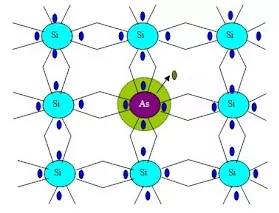(a) Frenkel defects                   (b) Schottky defects
(c) Impurity defects                 (d) n-type semiconductor.

47. Assertion: The total number of atoms present in a simple cubic unit cell is one.
Reason: Simple cubic unit cell has atoms at its corners, each of which is shared between eight adjacent unit cells.

48. If ‘a’ stands for edge length of cubic systems: body-centered and face-centered cubic then the ratio of the radii of the sphere in these systems will be respectively
(a) √3 a: a/√2                         (b) √3 a/2: √2 a/2
(c) √3 a/4: a/2√2                    (d) √3 a: √2 a

49. In a face-centered cubic unit cell, what is the volume occupied
(a) 4лr3 /3        (b) 8лr3 /3        (c) 16лr3 /3       (d) 64r3/3√3

50. An element crystallizing in the body-centered cubic lattice has an edge length of 500 pm. If its density is 4 g cm-3, the atomic mass of the element (in g mol-1) is (consider NA = 6 × 1023).
(a) 100             (b) 150                         (c) 200             (d) 250

Practice MCQs on Solid State Class 12 Chemistry

PRACTICE MCQS (ANSWERS) ON SOLID STATE CLASS 12 CHEMISTRY